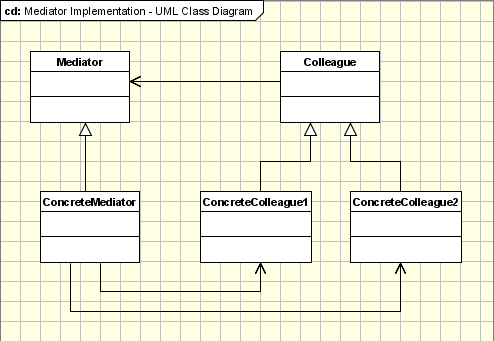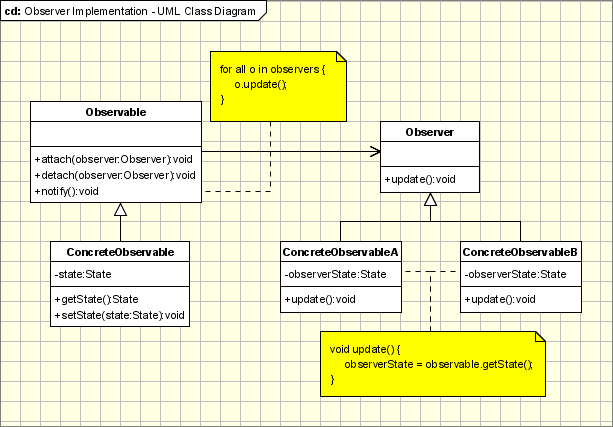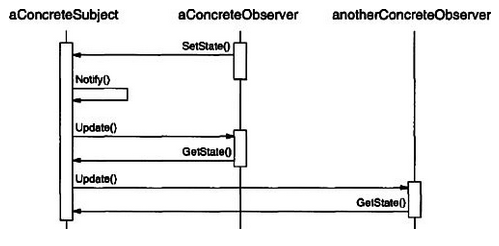# Introduction

Behavioral design patterns describe classes, objects, and communication between them to achieve complex flow control which is difficult to follow at run-time. The design patterns allow us to focus on the interaction of classes rather than on flow control. The behavioral patterns achieve the complex flow control via distributed responsibility using inheritance.

# Mediator

## Purpose

The Mediator design pattern describes a class (the Mediator) which defines the way in which two or more classes interact. This promotes loose coupling between the classes involved, removing the need for the various classes to explicitly refer to each other.

## Description

The interaction of classes is managed by a Mediator class. This is useful in situations where:

• A set of objects interact in a structured, but complex manner.
• Object reuse is difficult to achieve due to explicit references to other classes (tight coupling)
• Customizable interactions between multiple classes is to be achieved with minimal subclassing.

## Solution

The Mediator pattern has the following components:

1. Mediator: An abstract class which defines an interface for interacting with Colleague classes.
2. Concrete Mediator: Inherits from the Mediator and implements the collaborative behaviors between the Colleague classes.
3. Colleague Classes: The participant classes involved in the complex interactions via the Concrete Mediators.Each Colleague class contains a reference to the Mediator, with which it communicates rather than with other colleague classes.

Note: The Abstract Moderator class can be omitted if all Colleague classes interact only with a single Concrete Moderator.

## Python Implementation

Here is a template for the Mediator design pattern taken from the blog of Simon Wittber.

``````class Mediator(object):
def __init__(self):
self.signals = {}

def signal(self, signal_name, *args, **kw):
for handler in self.signals.get(signal_name, []):
handler(*args, **kw)

handlers = self.signals.setdefault(signal_name, [])

``````

# Observer

## Purpose

The Observer design pattern establishes a one-as-to-many relationship between classes, and ensures that when the Subject being observed changes state, all the Observers of the subject are notified of this change in state.

## Description

Rather than being limited to purely sequential code execution, the Observer pattern allows us to push notifications to classes based on events occurring. This decreases processor intensity, increases optimization, and increases efficiency.

## Solution

To achieve this event-based notification architecture, the Observer pattern makes use of the following components:

• Subject: An abstract class which defines an interface for attaching and detaching observers.
• Concrete Subject: Inherits from the Subject, and stores the state information which is of interest to the Concrete Observers, as well as the references to all observers to which it will send notifications when a state change occurs.
• Observer: An abstract class which defines an interface for objects to be notified on the change of state of a Subject being observed.
• Concrete Observer: A class which implements the Observer interface, and which handles the change of state notifications.In a typical scenario, an Observer will set the state of the Subject, which will the generate a notification, and send out a message to all Observers indicating that a state change has occurred. The Observers will then query the Subject for the new value(s).## Python Implementation

Below is a template which can be used for implementing the Observer pattern in Python:

``````from abc import ABCMeta, abstractmethod

class AbstractSubject:
__metaclass__ = ABCMeta

observers = []
state = 0

def __init__(self):
self.observers = []
self.state = 0

def register(self, observer):
self.observers.append(observer)
return self

def set_state(self, new_state):
self.state = new_state
self.notify()

def get_state(self):
return self.state

def notify(self):
for observer in self.observers:
observer.update()

class AbstractObserver:
__metaclass__ = ABCMeta

subject = 0

def __init__(self, obj):
self.subject = obj.register(self)

def set_state(self, new_state):
self.subject.set_state(new_state)

def update(self):
self.handle_state_change()

@abstractmethod
def handle_state_change(self):
raise NotImplementedError()

class Subject(AbstractSubject):

def show_state(self):
print "Subject: Current State = %s" %s (self.state)

class Observer(AbstractObserver):

old_state = 0
new_state = 0
id = 0

def set_id(self, id):
self.id = id

def handle_state_change(self):
self.old_state = self.new_state
self.new_state = self.subject.get_state()
print "Observer #%d: State Changed in Subject from '%s' to '%s'." % (self.id, self.old_state, self.new_state)

if __name__ == "__main__":
subject = Subject()

observer1 = Observer(subject)
observer1.set_id(1)

observer2 = Observer(subject)
observer2.set_id(2)

observer1.set_state("1 set by Observer 1")
observer2.set_state("2 set by Observer 2")
``````

# Strategy

## Purpose

The Strategy design pattern defines a class of encapsulated algorithms which are able to be used interchangeably. With the Strategy pattern, we can switch the algorithms independently from the client code.

## Description

The Strategy design pattern is used to decouple algorithmic implementations, including algorithm-specific data structures, from client code. When a class employs multiple conditional statements in determining behavior, it is a sign that you should consider making use of the Strategy design pattern to handle the complex behavior.

## Solution

The Strategy design pattern is made up of the following components:

• Strategy: An abstract class which defines the common interface for all supported algorithms.
• Concrete Strategy: A class inheriting from the Strategy class, and implementing the specific behavior (algorithm).
• Context: Utilizes the Strategy class to instantiate the appropriate Concrete Strategy to achieve the desired behavior.## Python Implementation

In the example below, we define an algorithm template in AbstractStrategy, which is then utilized by the various compatible strategies/algorithms, such as Addition, Subtraction, Multiplication and Division. Then, the Context is responsible for calling the behavioral function (strategy()) for the appropriate algorithm defined by the client.

``````from abc import ABCMeta, abstractmethod

class AbstractStrategy:
__metaclass__ = ABCMeta

@abstractmethod
def strategy(self, num_1, num_2):
raise NotImplementedError()

def strategy(self, num_1, num_2):
return num_1 + num_2

class Subtraction(AbstractStrategy):

def strategy(self, num_1, num_2):
return num_1 - num_2

class Multiplication(AbstractStrategy):

def strategy(self, num_1, num_2):
return num_1 * num_2

class Division(AbstractStrategy):

def strategy(self, num_1, num_2):
return num_1 / num_2

class Context:

strategy = 0

def __init__(self, strategy):
self.strategy = strategy

def calculate(self, num_1, num_2):
return self.strategy.strategy(num_1, num_2)

if __name__ == "__main__":
print context.calculate(10, 15)

context = Context(Subtraction())
print context.calculate(20, 5)

context = Context(Multiplication())
print context.calculate(12, 10)

context = Context(Division())
print context.calculate(12, 6)
``````

# Exercises

List at least three scenarios in which each of the following design patterns would be appropriate:

1. Mediator
2. Strategy
3. Observer

Continue to next section